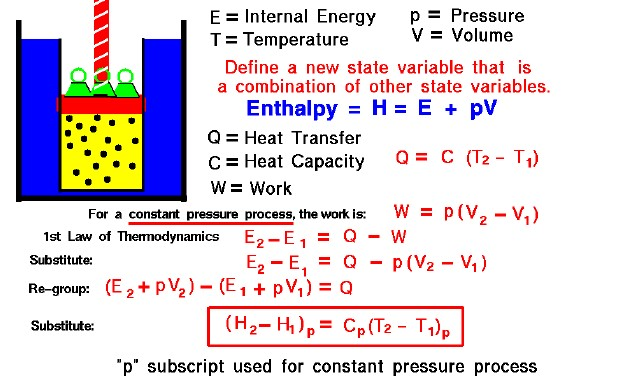# Enthalpy## Thermodynamics

Thermodynamics is a branch of physics which deals with the energy and work of a system. Thermodynamics deals only with the large scale response of a system which we can observe and measure in experiments. Like the Wright brothers, we are most interested in thermodynamics for the role it plays in engine design.

## State of a Gas

The state of a gas is defined by several properties including the temperature, pressure, and volume which the gas occupies. From our study of the first law of thermodynamics, we have found that the internal energy of a gas is also a state variable, that is, a variable which depends only on the state of the gas and not on any process that produced that state. We are free to define additional state variables which are combinations of existing state variables. These new variables will often make the analysis of a system much simpler. For a gas, a useful additional state variable is the enthalpy (H) which is defined to be the sum of the internal energy (E) plus the product of the pressure (p) and volume (V),

### H = E + p * V

How does one use this new variable called enthalpy? Let’s consider the first law of thermodynamics applied to a gas system with both heat transfer (Q) and work (W) done by the system in going from state 1 to state 2. From our slide on heat transfer, we know that we can represent the amount of heat transfer by a constant (C), called the heat capacity, times the difference in temperature (T):

### Q = C * (T2 – T1)

From our slide on the work done by a gas, we know two things. First, the amount of work depends on the process used to change the state. And second, that the work will be of the form pressure times the change of volume. Let us select a constant pressure process. Then the work is given by:

### W = p * (V2 – V1)

The first law of thermodynamics tells us:

### E2 – E1 = Q – W

Substitute the expression for the work:

### E2 – E1 = Q – p * [V2 – V1]

Let’s group the conditions at state 2 and the conditions at state 1 together:

### (E2 + p * V2) – (E1 + p * V1) = Q

The (E + p * V) can be replaced by the enthalpy (H)

### H2 – H1 = Q

Now substitute the value for “Q” which we talked about earlier:

### (H2 – H1)p = Cp (T2 – T1)p

We have enclosed the terms of the equation in parentheses with a “p” subscript to remind ourselves that this equation is only true for a constant pressure process.

#### What good is all of this?

The internal energy of a gas is hard to measure, but the temperature of a gas is easy to measure. Using the enthalpy for a gas lets us easily solve problems involving the first law of thermodynamics by measuring the temperature. The enthalpy is used in our derivation of the conservation of energy for a gas. We can apply the conservation of energy equation to determine the work performed during the various strokes of a four-stroke engine. The enthalpy is also used in our evaluation of the change of entropy as required by the second law of thermodynamics. Enthalpy is a very useful state variable when solving gas dynamics problems.

There is also a “specific” form of the enthalpy equation, which is just the derived form divided by the mass of the gas.

### (h2 – h1)p = cp (T2 – T1)p

The specific heat capacity (cp) is called the specific heat at constant pressure and is related to the universal gas constant of the equation of state. This final equation is used to determine values of specific enthalpy for a given temperature for a given gas. We can then use the tables of the results to solve problems.

Provide feedback